Next: Modifications for Al Up: Converting Al and Be Previous: Uncertainty in Be concentration

# Estimating the number of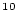Be atoms in the blank

The process blank protocol used in most chemical processing laboratories consists of processing one blank in parallel with each batch of 8-10 samples. As no sample is added to the process blank, the number ofBe atoms eventually found in it must come either from the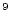Be carrier or from contamination during processing. By analogy with the discussion above, the number ofBe atoms in a particular process blank is: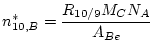(11)

Now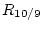is theBe/Be ratio measured in the process blank and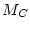is the mass of Be carrier used in the process blank. The other symbols are as used above. The superscripted star is intended to indicate that this is the number ofBe atoms in a particular blank, and not necessarily the summary value that should be used above in calculating the nuclide concentration in a particular sample (although it might be - see discussion below). The analytical uncertainty in the number ofBe atoms in the particular process blank is: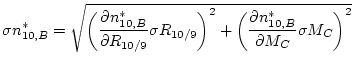(12)

where: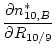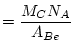(13)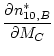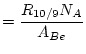(14)

Again,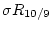is the uncertainty in the measuredBe/Be ratio of the process blank, and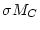is the uncertainty in the mass of Be carrier added to the process blank.

Having now calculated the number of atoms ofBe in a particular process blank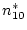, there are two ways to think about what blank value to use in determining the nuclide concentration in a sample, depending on what you believe about the source of theBe contamination in the laboratory.

One common way to think about this is to assume that all the samples in a particular batch are equally contaminated by unwantedBe atoms during processing. This would be true if these unwantedBe atoms came from sources that were common to all the samples in a batch. The simplest way to accomplish this would be if acids or other reagents were the source of the spuriousBe. In fact, the way we have set up this procedure, we know this to be true in part, because some (if not most) of the unwantedBe atoms come from the Be carrier, which is common to all the samples in the batch as well as the process blank that was processed along with them. This approach suggests that, when determining the nuclide concentration in a particular sample, theBe blank that should be taken into account is precisely the amount that was measured in the process blank run together with that sample. In this case, the values of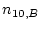and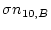that should be used in Equations 6 and 7 are the values of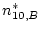and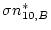calculated for the particular process blank that was run together in the same batch as your sample.

The second way to think about this is to assume that the number of atoms of unwantedBe in each sample is different. This would be the case if contamination came from soil particles, or other dust particles enriched in atmospherically producedBe that found their way into the lab; or if it came from cross-contamination between samples sometime during the chemical processing. If this were true, and we took the number ofBe atoms and their measurement uncertainty for a single blank as representative of the unwantedBe atoms in all samples, we would risk using a blank uncertaintythat was too small. The measurement uncertainty for a particular process blank would not take into account the fact that there might be a different number of unwantedBe atoms in the other samples in the batch. In this situation, it would be better to look at a series of process blanks run over a long period of time (as long as they were run using the same Be carrier solution). Then we would use the average and standard deviation of the long-term series of process blanks, instead of the value of any one blank, as the representative blank value and uncertainty to be applied to all of the samples run during this time.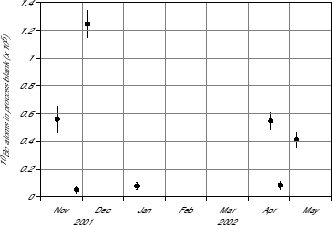Here's an example. Figure 1 shows a series of process blanks run over a 6-month period. All the process blanks contained the same amount of the same Be carrier, but the difference between blanks was much greater than the measurement precision of each individual blank. Eventually this problem was traced to unwantedBe atoms in the Al metal used to make the accelerator cathodes used to hold the Be samples for analysis, and it turned out that the number of spuriousBe atoms that turned up in the measurement was extremely variable between cathodes. This indicated that the number of unwantedBe atoms would be different for each sample, and that if we used the blank value and uncertainty measured for only the particular blank that was run in the same batch as a particular sample, we would underestimate the uncertainty in the sample nuclide concentration. Thus, for all the samples run during this period, it seemed best to use anandderived from the average and standard deviation of all these blanks (= 45000 and= 46000), instead of the measured value for any particular one of the blanks.Next: Modifications for Al Up: Converting Al and Be Previous: Uncertainty in Be concentration
2006-05-08